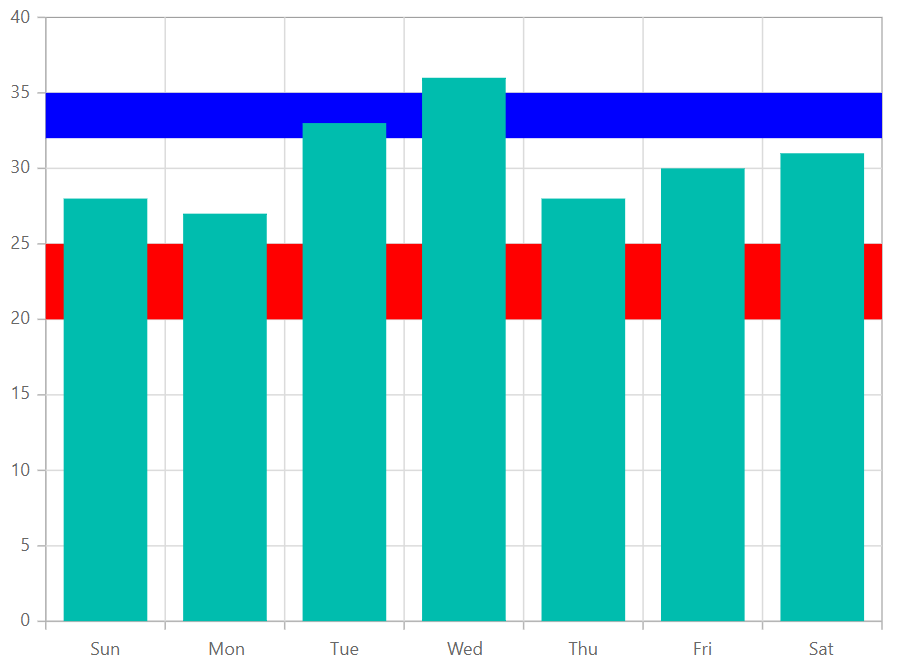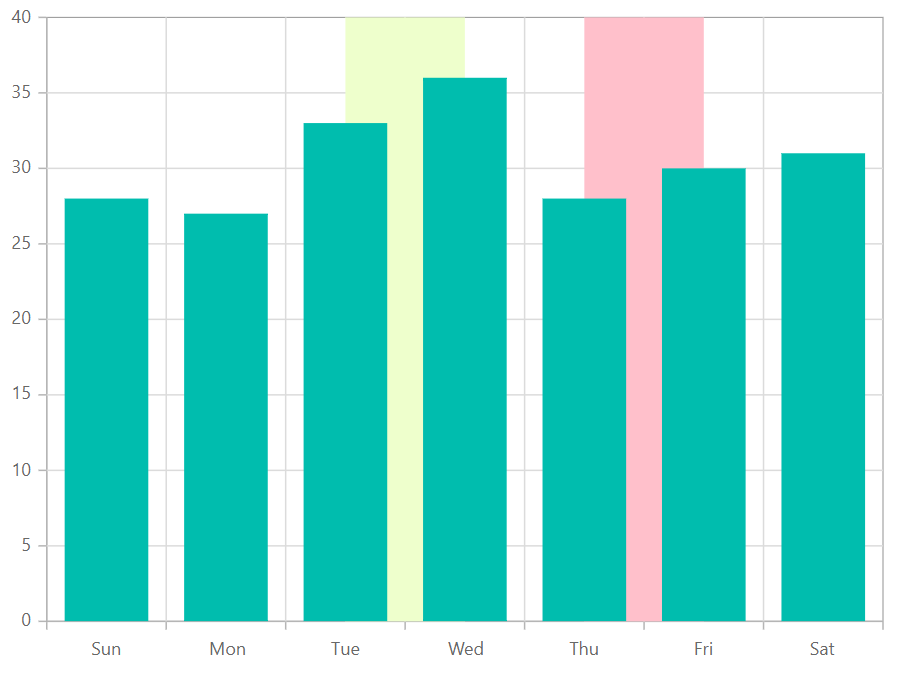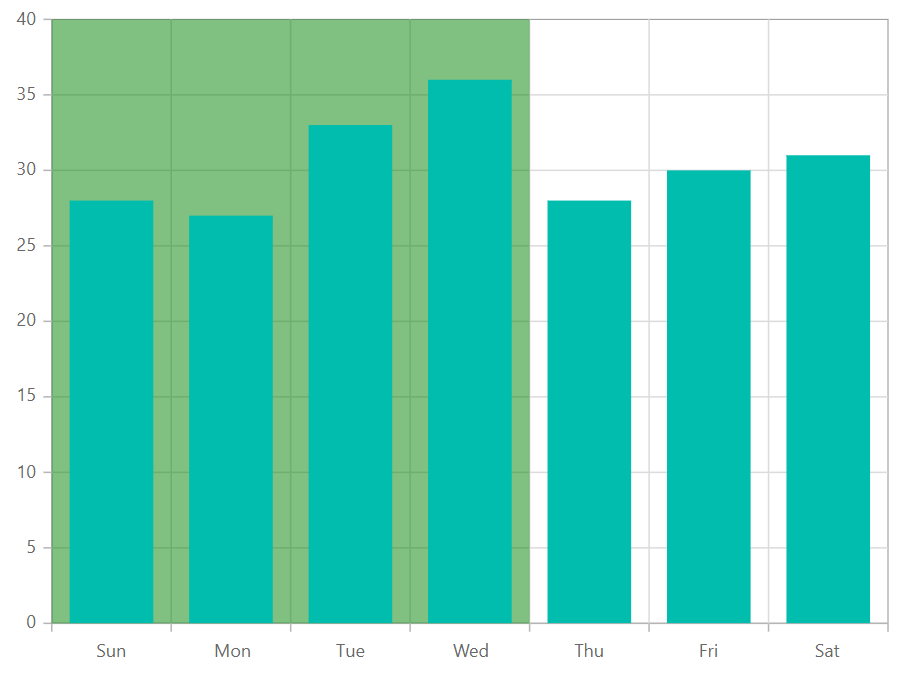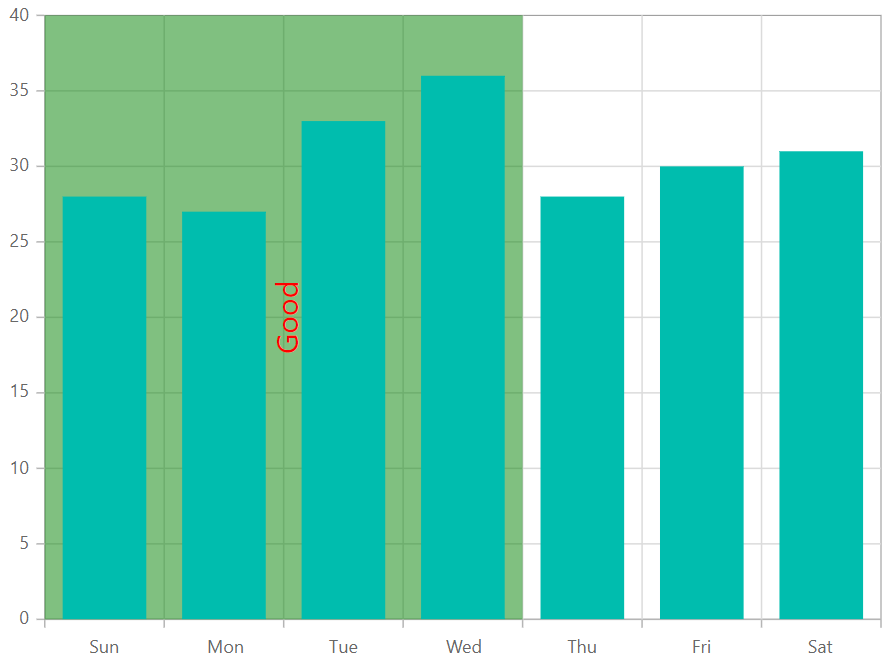Search results

# Stripline in Blazor Chart component

Blazor chart supports horizontal and vertical strip lines and customization of stripline in both orientation.

## Horizontal Striplines

You can create Horizontal stripline by adding the `StripLine` in the vertical axis. Striplines are rendered in the specified start to end range and you can add more than one stripline for an axis.

horizontal.razor
``````@using Syncfusion.Blazor.Charts

<SfChart>
<ChartPrimaryXAxis ValueType="Syncfusion.Blazor.Charts.ValueType.Category">
</ChartPrimaryXAxis>

<ChartPrimaryYAxis>
<ChartStriplines>
<ChartStripline Start="20" End="25" Color="red"></ChartStripline>
<ChartStripline Start="32" End="35" Color="blue"></ChartStripline>
</ChartStriplines>
</ChartPrimaryYAxis>

<ChartSeriesCollection>
<ChartSeries Type="ChartSeriesType.Column" DataSource="@WeatherReports" XName="X" YName="Y">
</ChartSeries>
</ChartSeriesCollection>

</SfChart>

@code{
public class ChartData
{
public string X;
public double Y;
}

public List<ChartData> WeatherReports = new List<ChartData>
{
new ChartData { X = "Sun", Y = 28 },
new ChartData { X = "Mon", Y = 27 },
new ChartData { X = "Tue", Y = 33 },
new ChartData { X = "Wed", Y = 36 },
new ChartData { X = "Thu", Y = 28 },
new ChartData { X = "Fri", Y = 30 },
new ChartData { X = "Sat", Y = 31 }
};
}``````## Vertical Striplines

You can create vertical stripline by adding the `StripLine` in the horizontal axis. Striplines are rendered in the specified start to end range and you can add more than one stripline for an axis.

vertical.razor
``````@using Syncfusion.Blazor.Charts

<SfChart>
<ChartPrimaryXAxis StripLines="@StripLines" ValueType="Syncfusion.Blazor.Charts.ValueType.Category">
</ChartPrimaryXAxis>

<ChartSeriesCollection>
<ChartSeries Type="ChartSeriesType.Column" DataSource="@WeatherReports" XName="X" YName="Y">
</ChartSeries>
</ChartSeriesCollection>
</SfChart>

@code{

public List<ChartStripline> StripLines = new List<ChartStripline>
{
new ChartStripline { Start= "2", End = "3", Color = "red" },
new ChartStripline { Start= "4", End = "5", Color = "blue" }
};

public class ChartData
{
public string X;
public double Y;
}

public List<ChartData> WeatherReports = new List<ChartData>
{
new ChartData { X = "Sun", Y = 28 },
new ChartData { X = "Mon", Y = 27 },
new ChartData { X = "Tue", Y = 33 },
new ChartData { X = "Wed", Y = 36 },
new ChartData { X = "Thu", Y = 28 },
new ChartData { X = "Fri", Y = 30 },
new ChartData { X = "Sat", Y = 31 }
};

}``````## Customize the strip line

Starting value in specific strip line can be customized by `Start` property in strip line. Similarly, ending value is customized by `End`. It can also set to start from the origin of the axis by `StartFromOrigin`. Size of the strip line can be customized by `Size` property. Border for the stripline can be customized by `Border` property. Order of the strip line such that whether it should be rendered behind or over the series elements can be customized by `ZIndex` property.

custom-stripline.razor
``````@using Syncfusion.Blazor.Charts

<SfChart>
<ChartPrimaryXAxis StripLines="@StripLines" ValueType="Syncfusion.Blazor.Charts.ValueType.Category">
</ChartPrimaryXAxis>

<ChartSeriesCollection>
<ChartSeries Type="ChartSeriesType.Column" DataSource="@WeatherReports" XName="X" YName="Y">
</ChartSeries>
</ChartSeriesCollection>
</SfChart>

@code{

public List<ChartStripline> StripLines = new List<ChartStripline>
{
new ChartStripline { Visible = true, StartFromAxis = true, Size = 4, ZIndex = ZIndex.Behind,
Opacity = 0.5, Color = "green" }
};

public class ChartData
{
public string X;
public double Y;
}

public List<ChartData> WeatherReports = new List<ChartData>
{
new ChartData { X = "Sun", Y = 28 },
new ChartData { X = "Mon", Y = 27 },
new ChartData { X = "Tue", Y = 33 },
new ChartData { X = "Wed", Y = 36 },
new ChartData { X = "Thu", Y = 28 },
new ChartData { X = "Fri", Y = 30 },
new ChartData { X = "Sat", Y = 31 }
};

}``````## Customize the stripline text

You can customize the text rendered in stripline by `TextStyle` property. Rotation of the text can be changed by `Rotation` property. Horizontal and Vertical alignment of stripline text can be changed by `HorizontalAlignment` and `VerticalAlignment` property.

custom-striptext.razor
``````@using Syncfusion.Blazor.Charts

<SfChart>
<ChartPrimaryXAxis StripLines="@StripLines" ValueType="Syncfusion.Blazor.Charts.ValueType.Category">
</ChartPrimaryXAxis>

<ChartSeriesCollection>
<ChartSeries Type="ChartSeriesType.Column" DataSource="@WeatherReports" XName="X" YName="Y">
</ChartSeries>
</ChartSeriesCollection>
</SfChart>

@code{

public List<ChartStripline> StripLines = new List<ChartStripline>
{
new ChartStripline { Visible = true, StartFromAxis = true, Size = 4, ZIndex = ZIndex.Behind,
Opacity = 0.5, Color = "green", Text= "Good", Rotation = 90, TextStyle = new ChartPrimaryYAxisStripLineFont{ Size = "15" },
VerticalAlignment = Anchor.Middle, HorizontalAlignment = Anchor.Middle }
};

public class ChartData
{
public string X;
public double Y;
}

public List<ChartData> WeatherReports = new List<ChartData>
{
new ChartData { X = "Sun", Y = 28 },
new ChartData { X = "Mon", Y = 27 },
new ChartData { X = "Tue", Y = 33 },
new ChartData { X = "Wed", Y = 36 },
new ChartData { X = "Thu", Y = 28 },
new ChartData { X = "Fri", Y = 30 },
new ChartData { X = "Sat", Y = 31 }
};

}``````